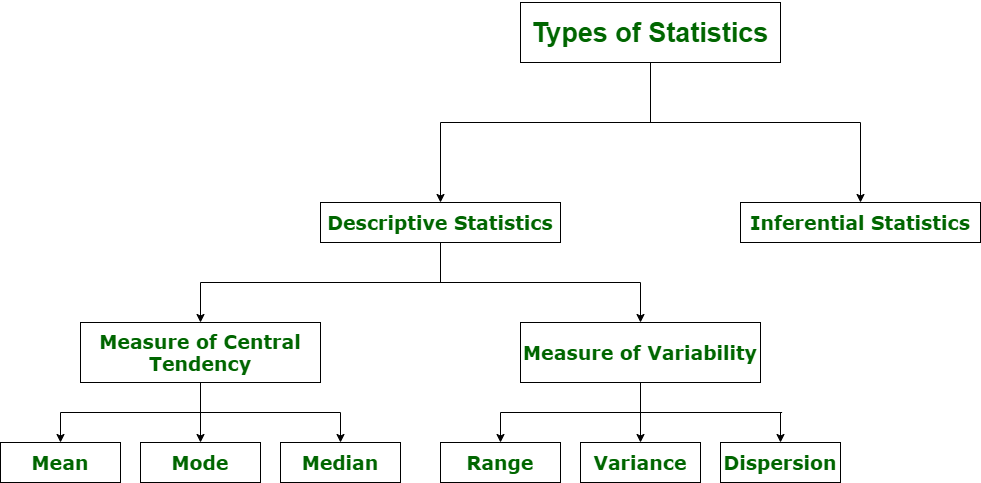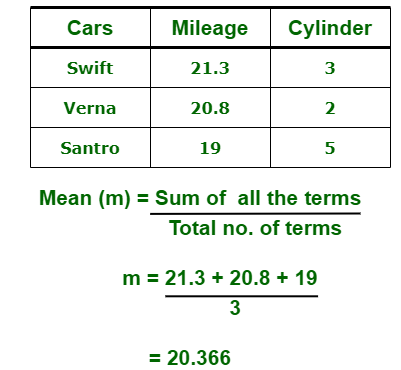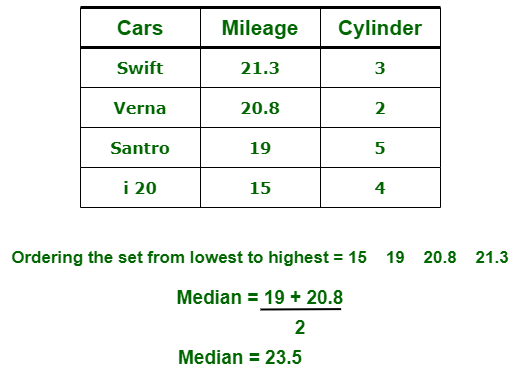# Introduction of Statistics and its Types

Statistics simply means numerical data, and is field of math that generally deals with collection of data, tabulation, and interpretation of numerical data. It is actually a form of mathematical analysis that uses different quantitative models to produce a set of experimental data or studies of real life. It is an area of applied mathematics concern with data collection analysis, interpretation, and presentation. Statistics deals with how data can be used to solve complex problems. Some people consider statistics to be a distinct mathematical science rather than a branch of mathematics.

Statistics makes work easy and simple and provides a clear and clean picture of work you do on a regular basis.

Basic terminology of Statistics :

• Population –
It is actually a collection of set of individuals or objects or events whose properties are to be analyzed.
• Sample –
It is the subset of a population.

Types of Statistics :1. Descriptive Statistics :
Descriptive statistics uses data that provides a description of the population either through numerical calculation or graph or table. It provides a graphical summary of data. It is simply used for summarizing objects, etc. There are two categories in this as following below.

• (a). Measure of central tendency –
Measure of central tendency is also known as summary statistics that is used to represents the center point or a particular value of a data set or sample set.
In statistics, there are three common measures of central tendency as shown below:

• (i) Mean :
It is measure of average of all value in a sample set.
For example,• (ii) Median :
It is measure of central value of a sample set. In these, data set is ordered from lowest to highest value and then finds exact middle.
For example,• (iii) Mode :
It is value most frequently arrived in sample set. The value repeated most of time in central set is actually mode.
For example,• (b). Measure of Variability –
Measure of Variability is also known as measure of dispersion and used to describe variability in a sample or population. In statistics, there are three common measures of variability as shown below:

• (i) Range :
It is given measure of how to spread apart values in sample set or data set.

`Range = Maximum value - Minimum value `
• (ii) Variance :
It simply describes how much a random variable defers from expected value and it is also computed as square of deviation.

`S2= ∑ni=1 [(xi - ͞x)2 ÷ n]    `

In these formula, n represent total data points, ͞x represent mean of data points and xi represent individual data points.

• (iii) Dispersion :
It is measure of dispersion of set of data from its mean.

`σ= √ (1÷n) ∑ni=1 (xi - μ)2  `

2. Inferential Statistics :
Inferential Statistics makes inference and prediction about population based on a sample of data taken from population. It generalizes a large dataset and applies probabilities to draw a conclusion. It is simply used for explaining meaning of descriptive stats. It is simply used to analyze, interpret result, and draw conclusion. Inferential Statistics is mainly related to and associated with hypothesis testing whose main target is to reject null hypothesis.

Hypothesis testing is a type of inferential procedure that takes help of sample data to evaluate and assess credibility of a hypothesis about a population. Inferential statistics are generally used to determine how strong relationship is within sample. But it is very difficult to obtain a population list and draw a random sample.

Inferential statistics can be done with help of various steps as given below:

2. Generate a research hypothesis.
3. Operationalize or use variables
4. Identify or find out population to which we can apply study material.
5. Generate or form a null hypothesis for these population.
6. Collect and gather a sample of children from population and simply run study.
7. Then, perform all tests of statistical to clarify if obtained characteristics of sample are sufficiently different from what would be expected under null hypothesis so that we can be able to find and reject null hypothesis.

Types of inferential statistics –
Various types of inferential statistics are used widely nowadays and are very easy to interpret. These are given below:

• One sample test of difference/One sample hypothesis test
• Confidence Interval
• Contingency Tables and Chi-Square Statistic
• T-test or Anova
• Pearson Correlation
• Bi-variate Regression
• Multi-variate Regression

Attention reader! Don’t stop learning now. Get hold of all the important CS Theory concepts for SDE interviews with the CS Theory Course at a student-friendly price and become industry ready.

My Personal Notes arrow_drop_upCheck out this Author's contributed articles.

If you like GeeksforGeeks and would like to contribute, you can also write an article using contribute.geeksforgeeks.org or mail your article to contribute@geeksforgeeks.org. See your article appearing on the GeeksforGeeks main page and help other Geeks.

Please Improve this article if you find anything incorrect by clicking on the "Improve Article" button below.

Article Tags :

1

Please write to us at contribute@geeksforgeeks.org to report any issue with the above content.